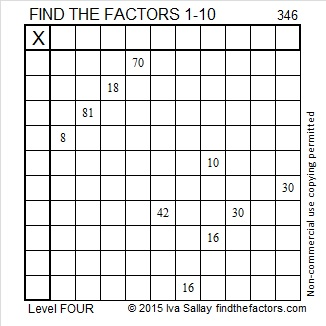# 346 and Level 4

Quick Prime/Composite Number Test: 346 is even and thus divisible by 2, so 346 is a composite number.Print the puzzles or type the factors on this excel file: 10 Factors 2015-01-05

• 346 is a composite number.
• Prime factorization: 346 = 2 x 173
• The exponents in the prime factorization are 1 and 1. Adding one to each and multiplying we get (1 + 1)(1 + 1) = 2 x 2 = 4. Therefore 346 has exactly 4 factors.
• Factors of 346: 1, 2, 173, 346
• Factor pairs: 346 = 1 x 346 or 2 x 173
• 346 has no square factors that allow its square root to be simplified. √346 ≈ 18.601This site uses Akismet to reduce spam. Learn how your comment data is processed.